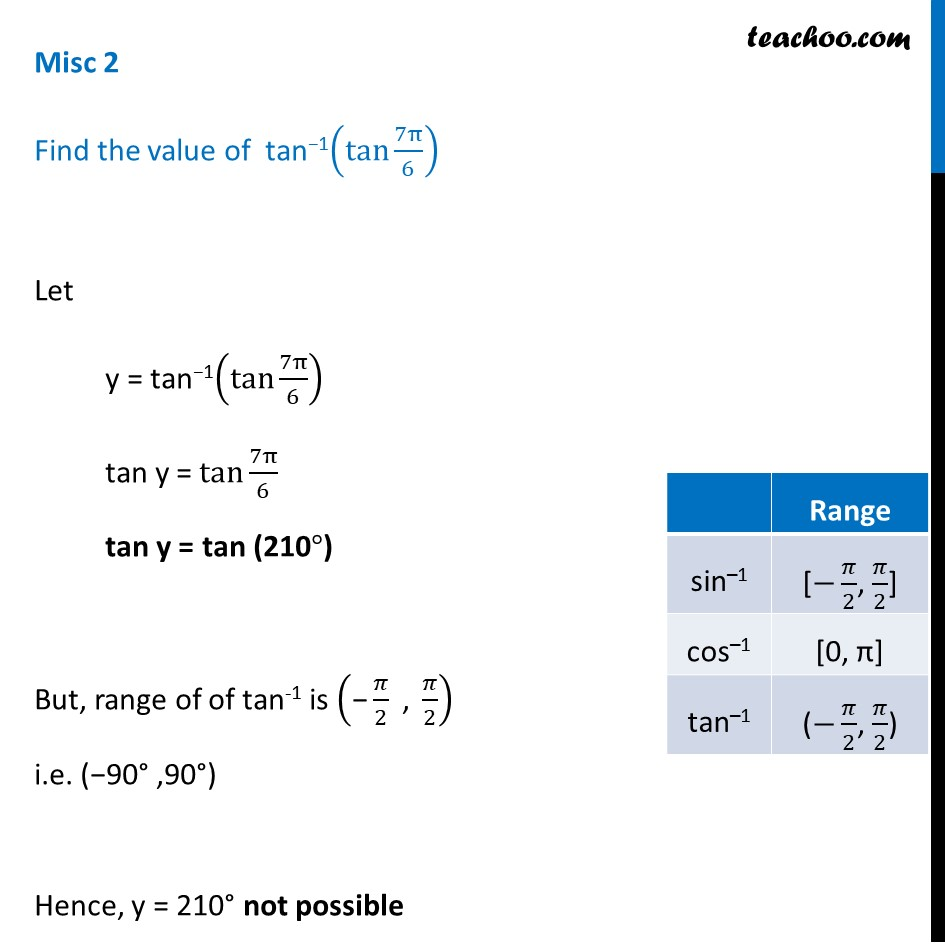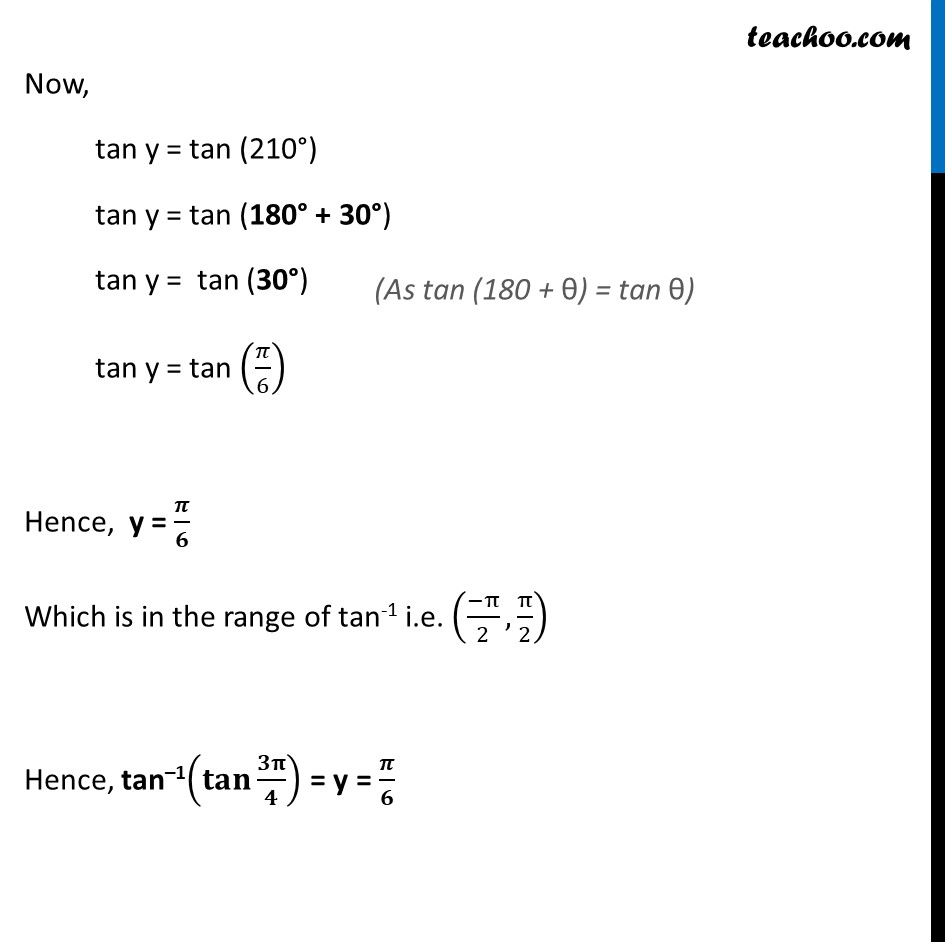Chapter 2 Class 12 Inverse Trigonometric Functions

Class 12
Important Questions for exams Class 12Learn in your speed, with individual attention - Teachoo Maths 1-on-1 Class

### Transcript

Misc 2 Find the value of tan−1(tan⁡〖7π/6〗 ) Let y = tan−1(tan⁡〖7π/6〗 ) tan y =〖 tan〗⁡〖7π/6〗 tan y = tan (210°) But, range of of tan-1 is ("−" 𝜋/2 " , " 𝜋/2) i.e. (−90° ,90°) Hence, y = 210° not possible Now, tan y = tan (210°) tan y = tan (180° + 30°) tan y = tan (30°) tan y = tan (𝜋/6) Hence, y = 𝝅/𝟔 Which is in the range of tan-1 i.e. ((−π)/2, π/2) Hence, tan–1(𝐭𝐚𝐧⁡〖𝟑𝛑/𝟒〗 ) = y = 𝝅/𝟔 (As tan (180 + θ) = tan θ)